# How to add leading zeros in R, Power Query, DAX or ExcelHere are multiple examples of how to add leading zeros in different ways and places. In these examples, I will add them to number 123. As a result, I will get 5 digits (00123).

In R there are more than two options on how to add leading zeros. Here are two of them that work with base functions sprintf and formatC.

`sprintf("%05d", 123)`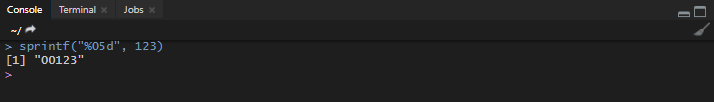`formatC(x = 123, width = 5, flag = 0)`In Power Query that is not so easy, because you have to combine two functions Text.PadStart and Text.From.

`Text.PadStart(Text.From([Without leading zeros]), 5, "0")`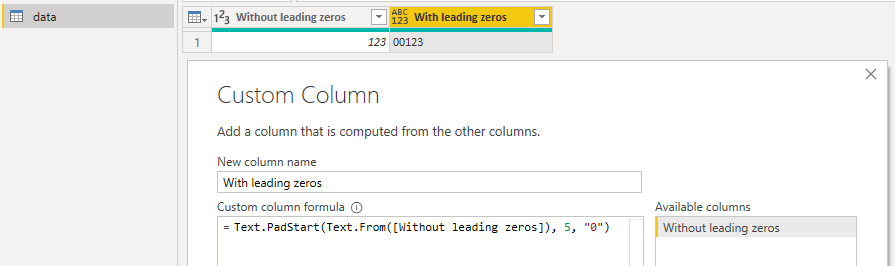## Replace Power Query column values with the function result

With this approach, you can add leading zeros in Power Query without extra calculated column and you can use this technique in other, similar cases.

It is easier to start with some framework. Right-click on the column and make some replacing that generates the necessary function.In the replace step, change the old value and new value from this…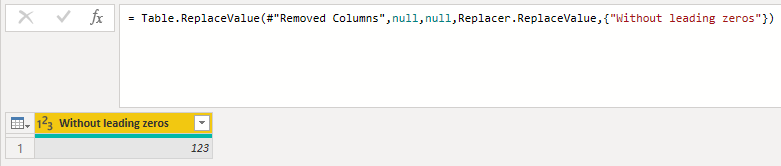to this…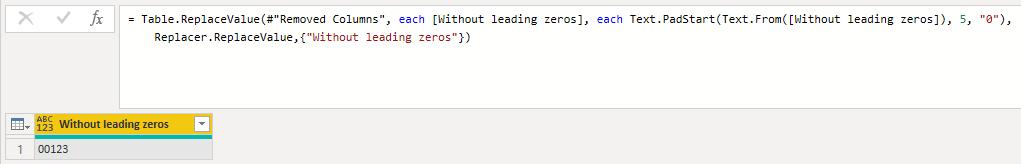`= Table.ReplaceValue(#"Removed Columns", each [Without leading zeros], each Text.PadStart(Text.From([Without leading zeros]), 5, "0"), Replacer.ReplaceValue,{"Without leading zeros"})`

In DAX this is similar to Excel. But instead of Excel function TEXT, you should use function FORMAT where “00000” in this case works like Excel format code. Every 0 defines the minimum amount of digits.

`With leading zeros = FORMAT(data[Without leading zeros]; "00000")`To do that you have to be a little bit familiar with formating code. You can see some of the format code examples in format cells (Ctrl +1) window and category Custom.

For example, format code 00 means that in the result is at least 2 digits and zero is added if you have only 1 digit. In other words, 0 means each required digit. By using formatting code you can add them only visually or permanently.

### Permanently with TEXT function

In cell A1 you have 123 and you need 5 digits and, in this case, additional zeros.

`=TEXT(A1;"00000")`### Only visually with Format Cells and Custom format

Open Format Cells window with Ctrl + 1 and add format code, where every 0 defines every displayed digit.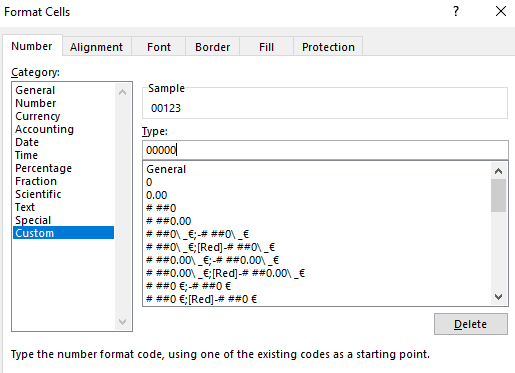Here is the result. As you see in the formula bar, the result is only visual.If you are an experienced Excel user and would try to use R, check out this post.